# Classification of Machine Learning Models

In recent days, Machine Learning is showing tremendous potential but compared to human intelligence, it is still in its earliest stage and specialized to the problem for which it is developed. For example, any ML model developed to predict cancer cells will not predict the presence of Cats in those images. In the current stage, it is not able to observe multiple dimensions and gain intelligence from there. Machine Learning can learn only those specific things for which it is specially designed. So we can say that it is controlled by humans who have designed them.

A group of scientists is also researching Artificial General Intelligence (AGI) related to self-decision-making machines. The concept of AGI is too complex to discuss here.

At the current stage, we can say that any Machine Learning approach can be mapped to find interdependencies or relations between input and output data.

If we try to find the answer to Different types of Machine Learning, we will get different answers, like Classification and Regression, Supervised and Unsupervised, Probabilistic and Non-probabilistic, and many more.

BUT! Have you ever thought?

### Why are there different classification categories for the same Machine Learning?

To find this answer, let’s quickly see the simplest pipeline for any machine learning approach to solve the given problem statement.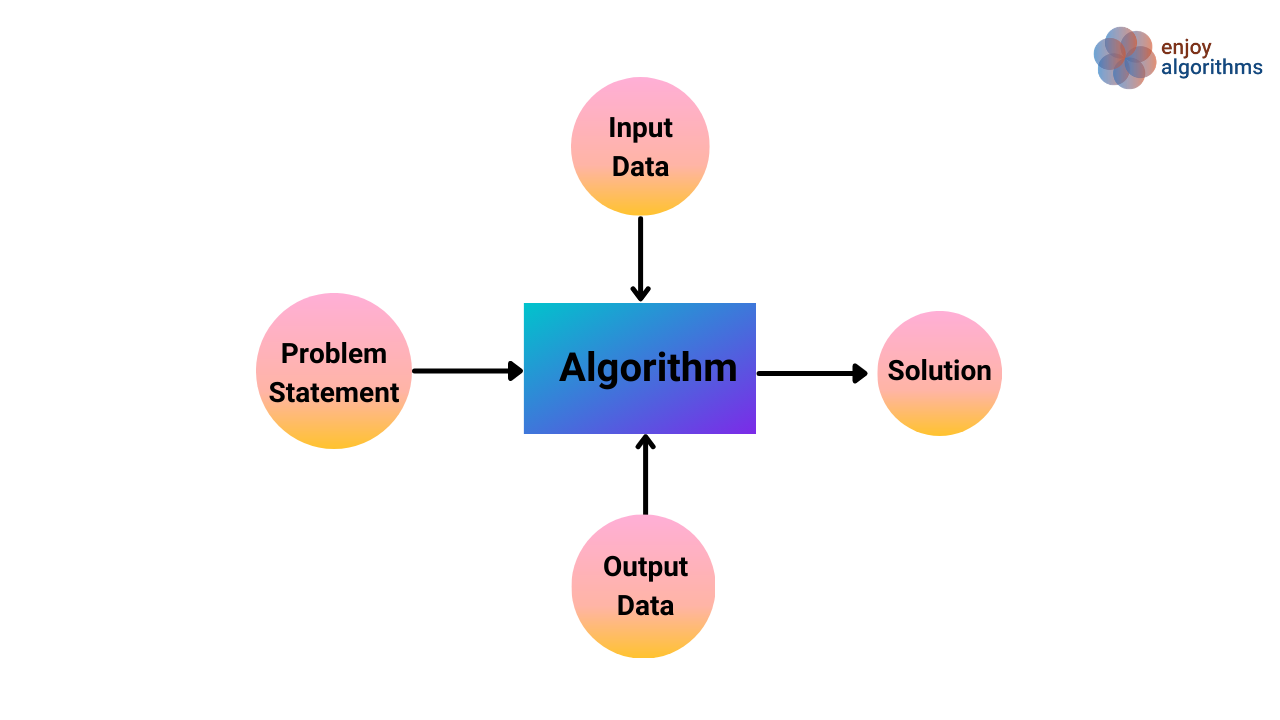There are 5 major components playing a vital role in the whole pipeline and the classification of Machine Learning depends upon the nature of all these 5 components. Looking into this pipeline, we can quickly identify the 5 different bases on which Machine Learning can be classified.

1. Nature of Input Data
2. Nature of Problem
3. Nature of Algorithm
4. Nature of Solution
5. Nature of Output Data

Let’s start classifying it on every basis one by one.

### Classification Based on Nature of Inputs

Based on the type of Input data that is used to train the algorithms, Machine Learning problems can be classified into four different categories,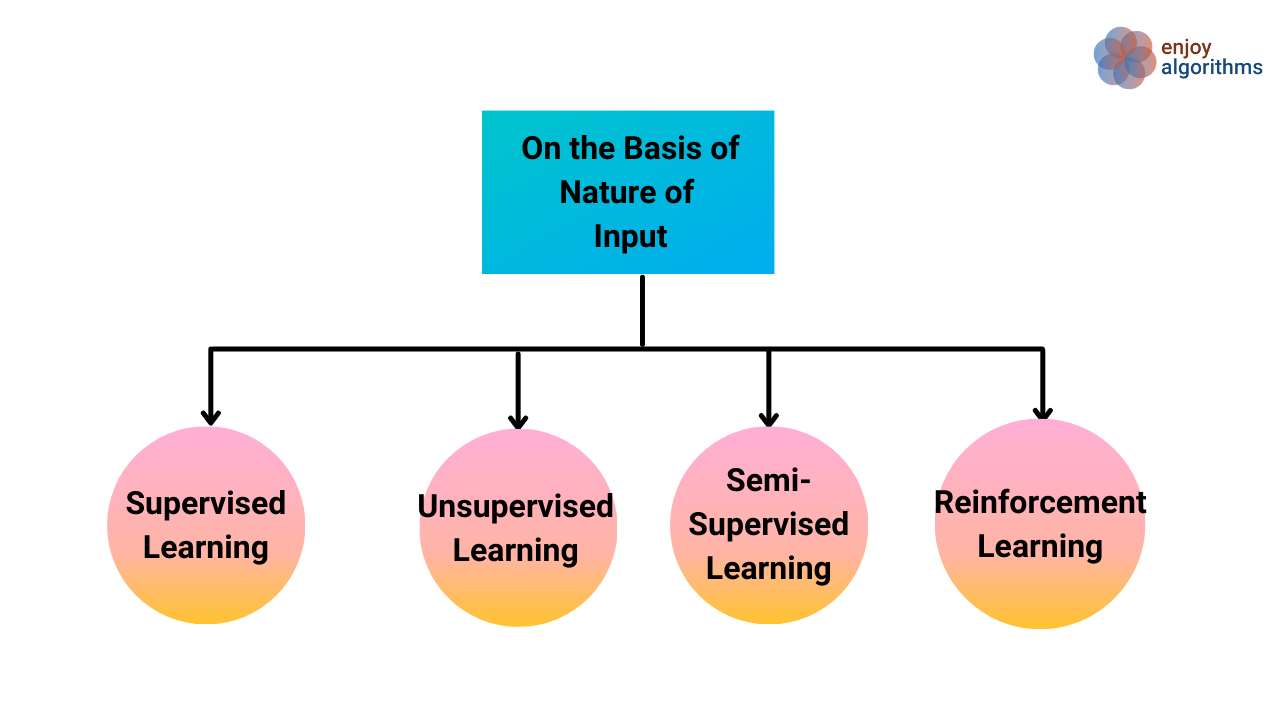Let's explore each of these categories in detail

1. Supervised Learning:- Supervised learning is where we have input variables (X) and an output variable (Y). We use a machine-learning algorithm to learn the mapping function from the input to the output.

It is called Supervised learning because the process of learning from training data can be thought of as a teacher who is supervising the learning process. In the below figure, the teacher explicitly provides information on the input data in the form of annotation.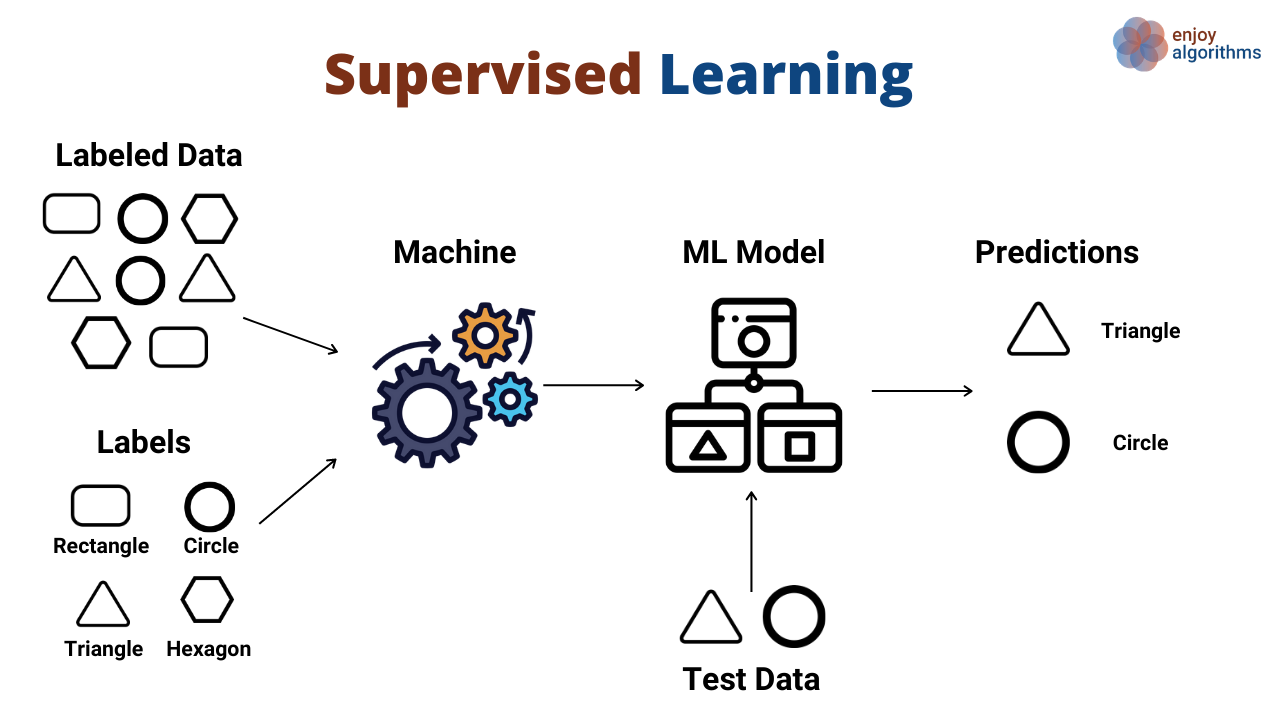1. Unsupervised Learning:- Here we only have input data (X) and no corresponding output variables. So there is no teacher to supervise. But now we must be thinking, how machines will learn mapping functions then? This is an interesting field, where machines try to form a pseudo output and then learn mapping functions. Pseudo output can be like the similarity among data samples. In simple terms, we can say that they try to treat underlying characteristics present in the input data as their output data. No explicit output data is required.

This approach is mainly used to dive deeper into data analysis. If you compare this with the above image in the image below, we don’t have any information about the shape type of the input data. But our model can still segregate them based on their color and size. This segregation, done by machines, can be termed Unsupervised Learning.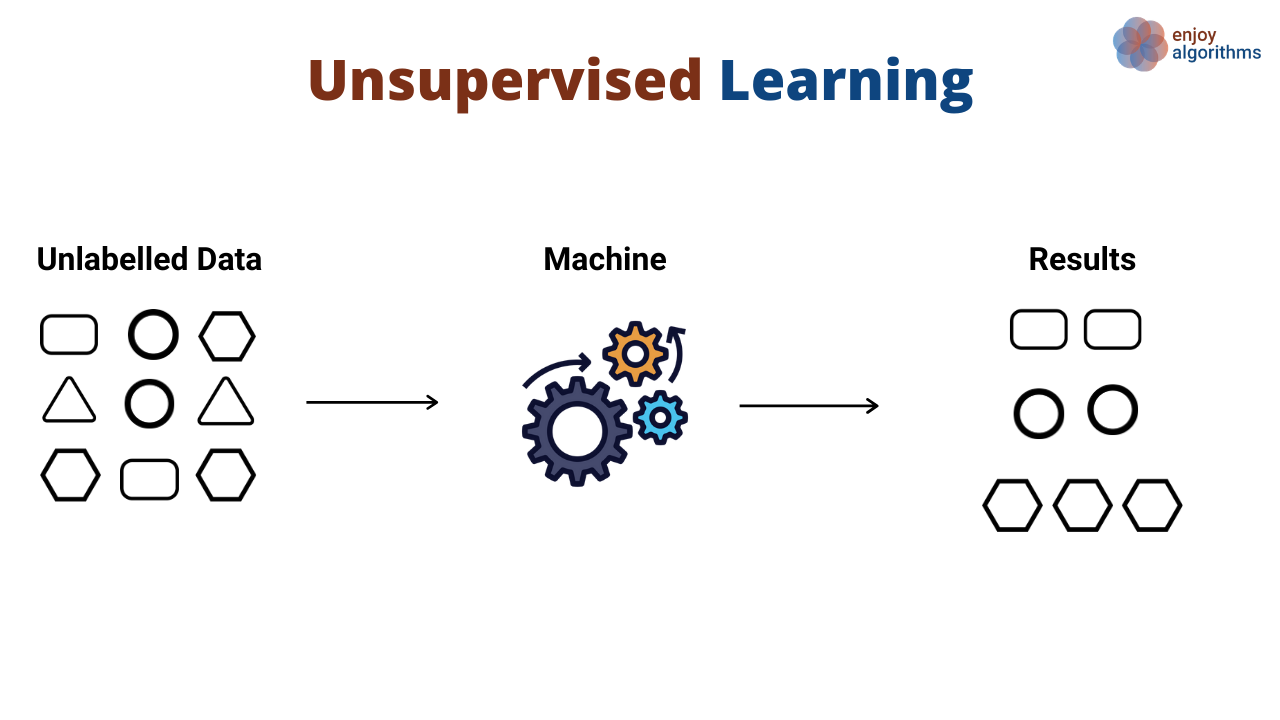1. Semi-Supervised Learning:- Problems where we have a large amount of input data (X), and only some of the data is annotated (Y) are called semi-supervised learning problems. e.g., A large dataset of images in which some are annotated (labeled), and some are not. In the above image, suppose in input data, explicit annotation about the circle and triangle is given, but for rectangle and hexagon, it is missing. This scenario will fall under the category of Semi-Supervised Learning.

Most of the real-world data lie in this category as labeling data is time-consuming and requires expert human resources.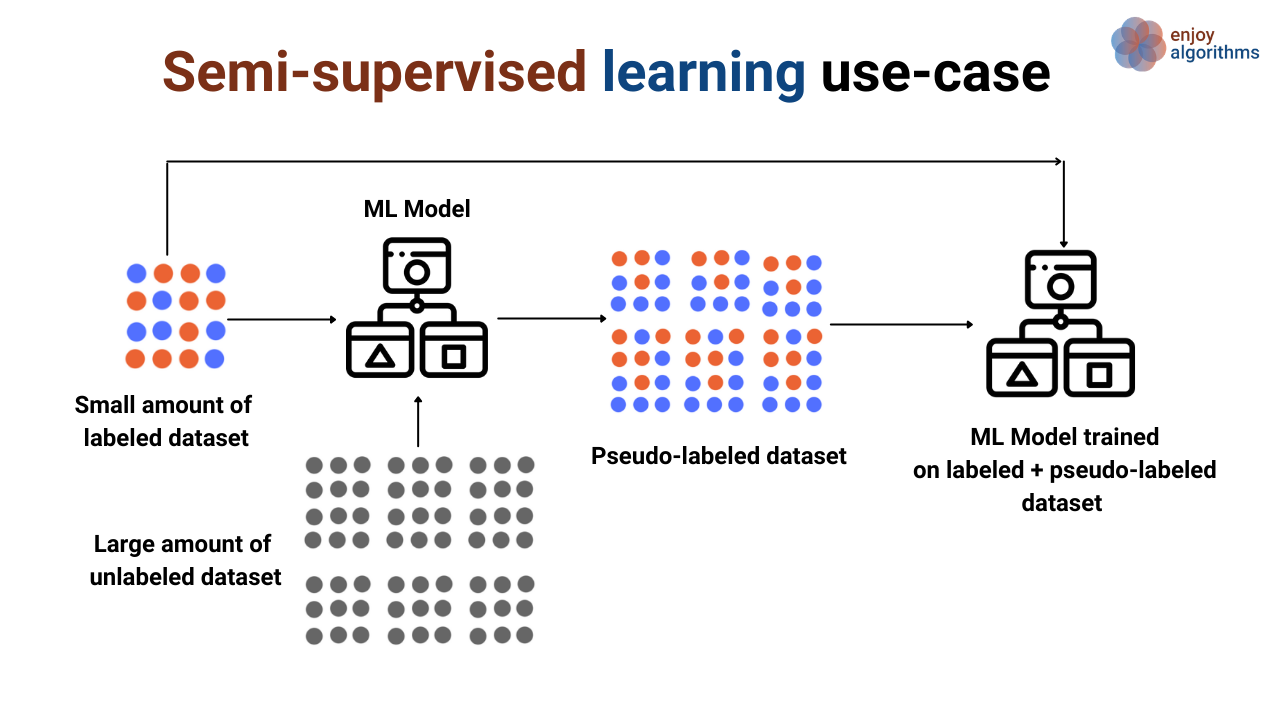1. Reinforcement Learning (RL):- In supervised learning, we present our data in pairs of input and output to our algorithms. In Reinforcement Learning, our machines work as an agent in a virtual environment and perform possible actions in that environment. For example, suppose we asked our machines to play chess. Here Machine is the agent, and the chessboard is the environment.

Our agent is trying to move King, and we know that the king can perform specific movements only. So all the movements a king can make at any stage of the game is the possible set of actions that our agent can take. The agent selects the best action from all the options present at that environmental state, and based on that selection, receives reward/risks. In simple words, our agent will not take any such move that will land our king in checkmate condition. The algorithm tries to maximize the reward with the best set of actions or minimize the risk (of losing the game), and in this way, it learns.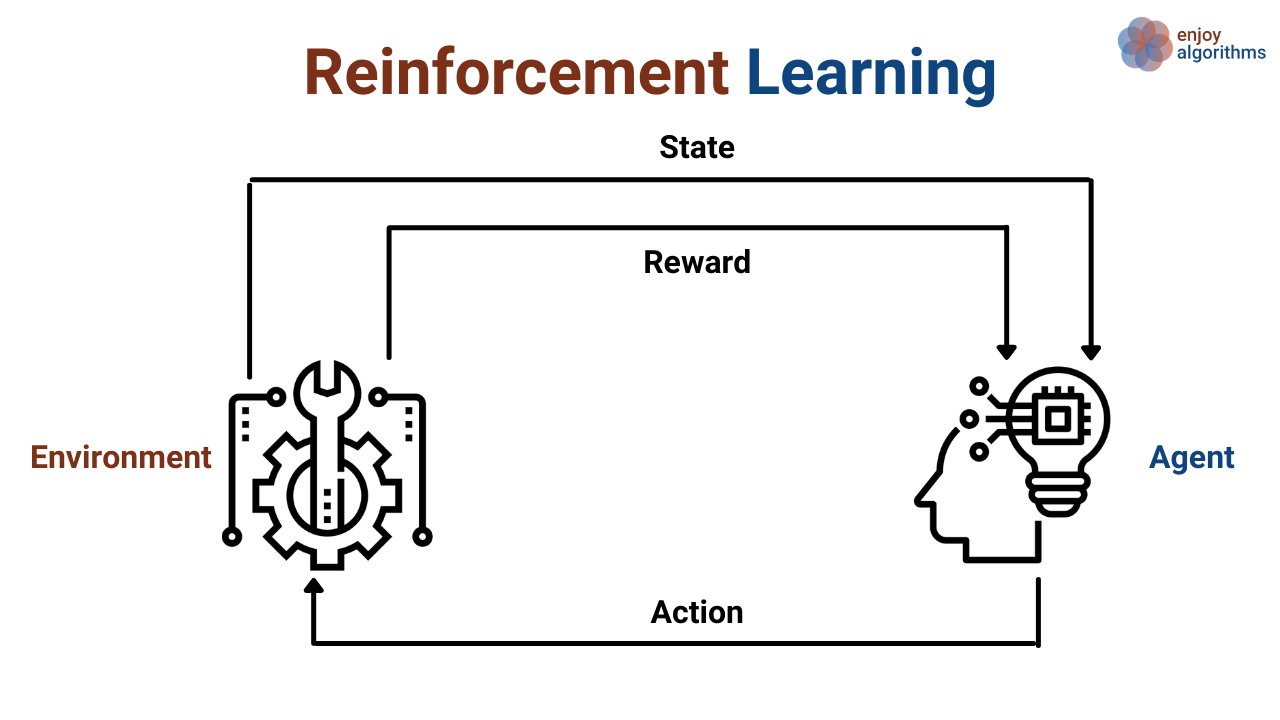### Classification Based on Nature of the Problem

Based on the type of problem that we are trying to solve, we can classify the Machine learning problem into three different categories.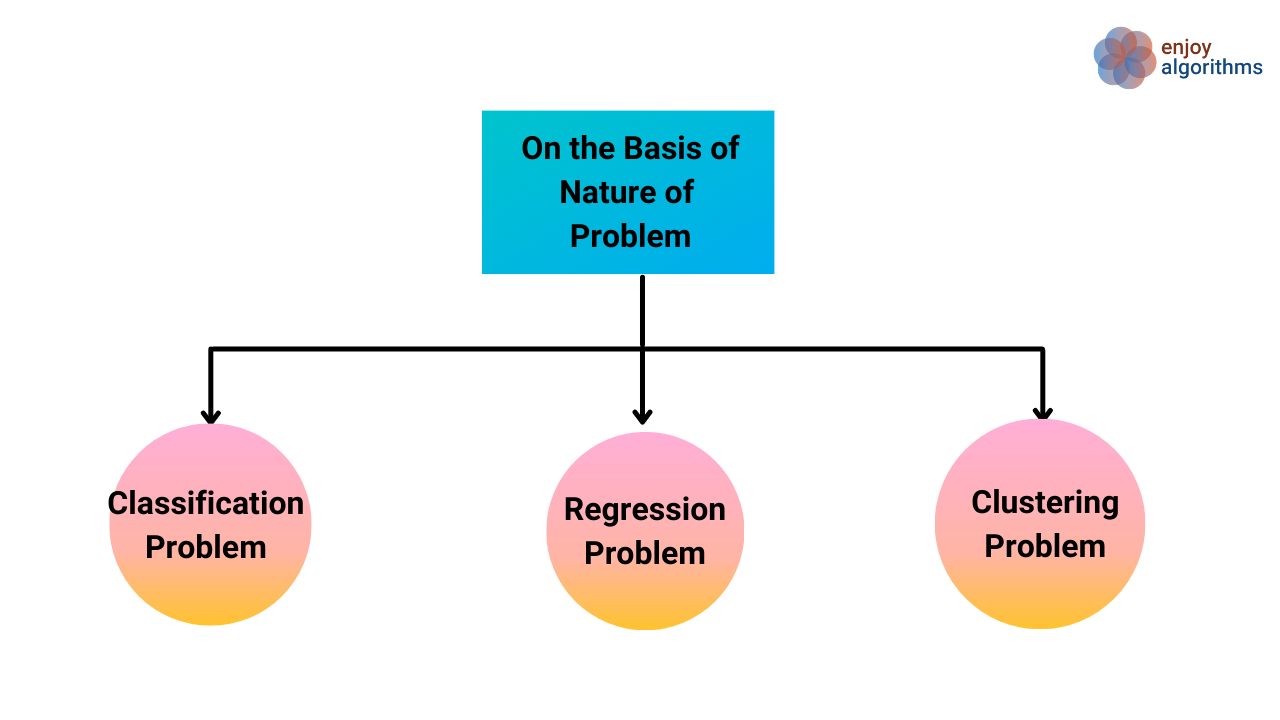Let's dive deeper into each of these categories

1. Classification Problem:- Classification is a problem that requires machine learning algorithms that learn how to assign a class label to examples from the problem domain. A very intuitive example is classifying images into two labels “Dog” or “Not A Dog.”
2. Regression Problem:- Regression is a problem that requires machine learning algorithms that learn to predict continuous variables. An elementary example will be to predict the temperature of the city. (Temperature can take any numeric value between -50 to +50 degrees Celsius.)
3. Clustering Problem:- Clustering is a type of problem that requires the use of Machine Learning algorithms to group the given data samples into a specified number of groups. A simple example will be to group the lemons according to their sizes.
Note:- It apparently resembles the classification problem, but the major difference between these algorithms is, the Classification problem is Supervised Learning while Clustering is Unsupervised Learning.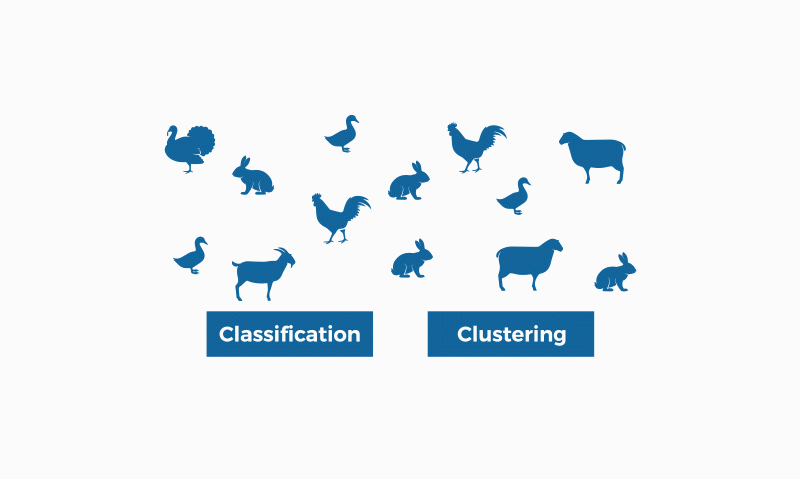### Classification Based on Nature of Algorithm :

Based on the Nature of the Algorithm used in the Machine Learning process, Machine learning can be classified into three categories.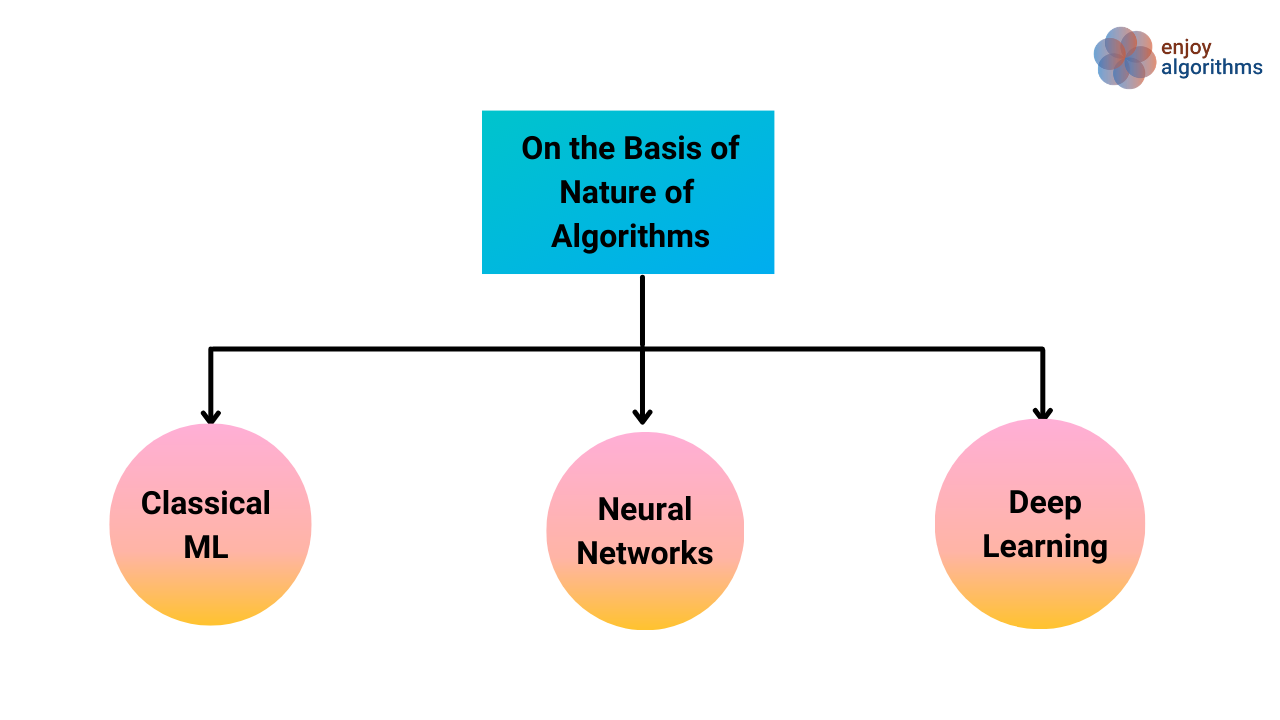1. Classical Machine Learning:- Algorithms that use Statistical and Mathematical equations to derive the relations in input and output data, come under this category. These algorithms are also called Statistical Machine Learning algorithms. It has the advantage of explain-ability (the ability to explain the reason for certain predictions for the given input). e.g., K-means, Decision Trees, Random Forest, Support Vector Machine (SVM), Linear Regression, etc.
2. Neural Networks:- Algorithms that are inspired by human brains. In the process of these algorithms, a complex mathematical model with a large number of trainable parameters ( entries of weight and bias matrices) is built. These parameters are trained by using training data. Neural networks seem quite promising but face a lot of limitations when the complexity of the model increases. It also has a limitation in capturing complex dependencies like temporal/spatial dependencies. Temporal dependencies are the dependencies that depend on time (how the input sample at time step t1 depends upon the input sample at time step t2), and Spatial dependencies are dependencies that depend upon space (how input collected at one condition depends upon input at different conditions).
3. Deep-Learning:- Basic principle of Deep-learning is the same as Neural Networks, but some advancement in terms of the hidden layers' placement (architecture) is introduced to tackle Neural Networks' limitations. Deep-learning algorithms are capable of learning Spatial/Temporal relations in training data. But the major drawback with these algorithms is non-explainability.
Nowadays, in industries, Explainable AI is in high demand. Examples of these algorithms are Convolutional Neural Networks (CNNs), Recurrent Neural Networks (RNNs), Long Short-Terms Memory (LSTM), etc.

### Classification Based on Nature of Solution

Based on solution nature, one can classify machine learning into two different categories.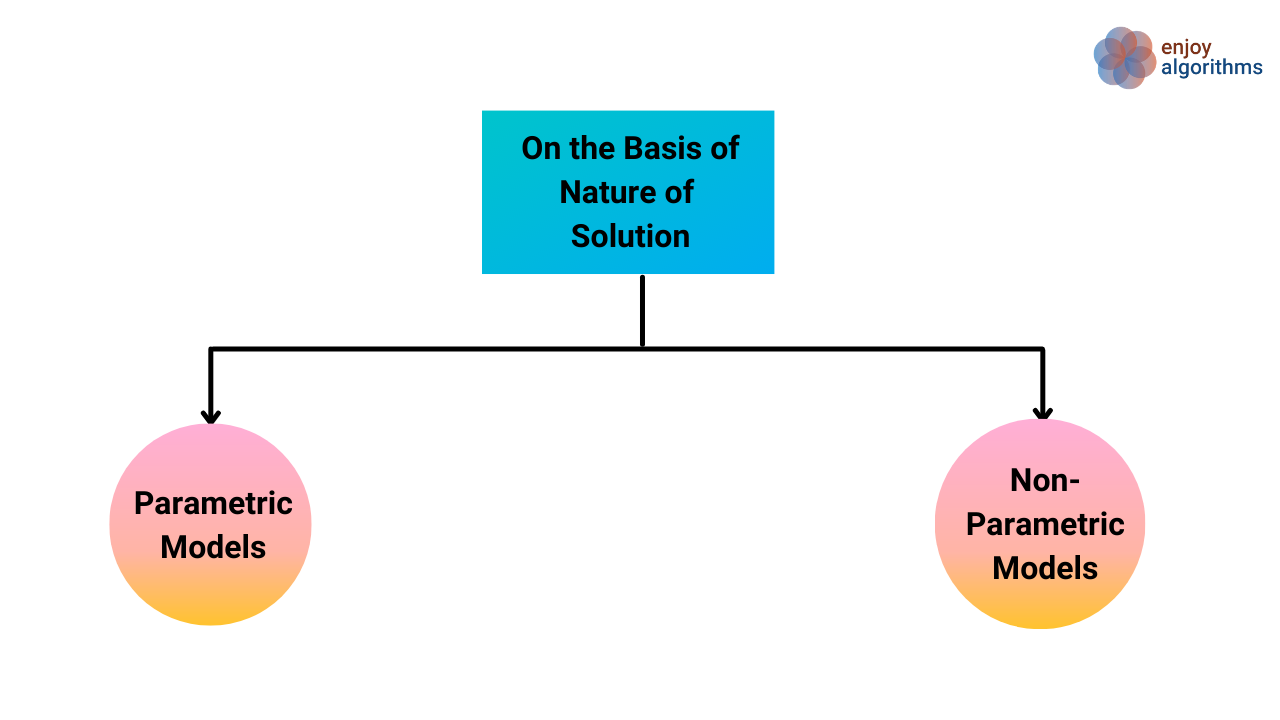Naturally, ML algorithms are designed to learn the historical input data, make inferences from that historical data, and predict the output for future inputs.
To predict the output, the model can take two approaches:-

1. Parametric Models:- Models that consider only future inputs to predict the output. Such models take the analogy from the training data and expect the same analogy to be followed in the unseen testing data. Linear regression and Neural Networks are examples of parametric models.
2. Non- Parametric Models:- Prediction of output depends on the Input features and previous outputs that the model has predicted earlier. In this approach, the predicted output value is derived from the output values in similar scenarios identified from training data. KNN and Decision Trees are examples of non-parametric models.

### Classification Based on Nature of Output Data

Based on the Nature of output, Machine learning can be classified into two different categories.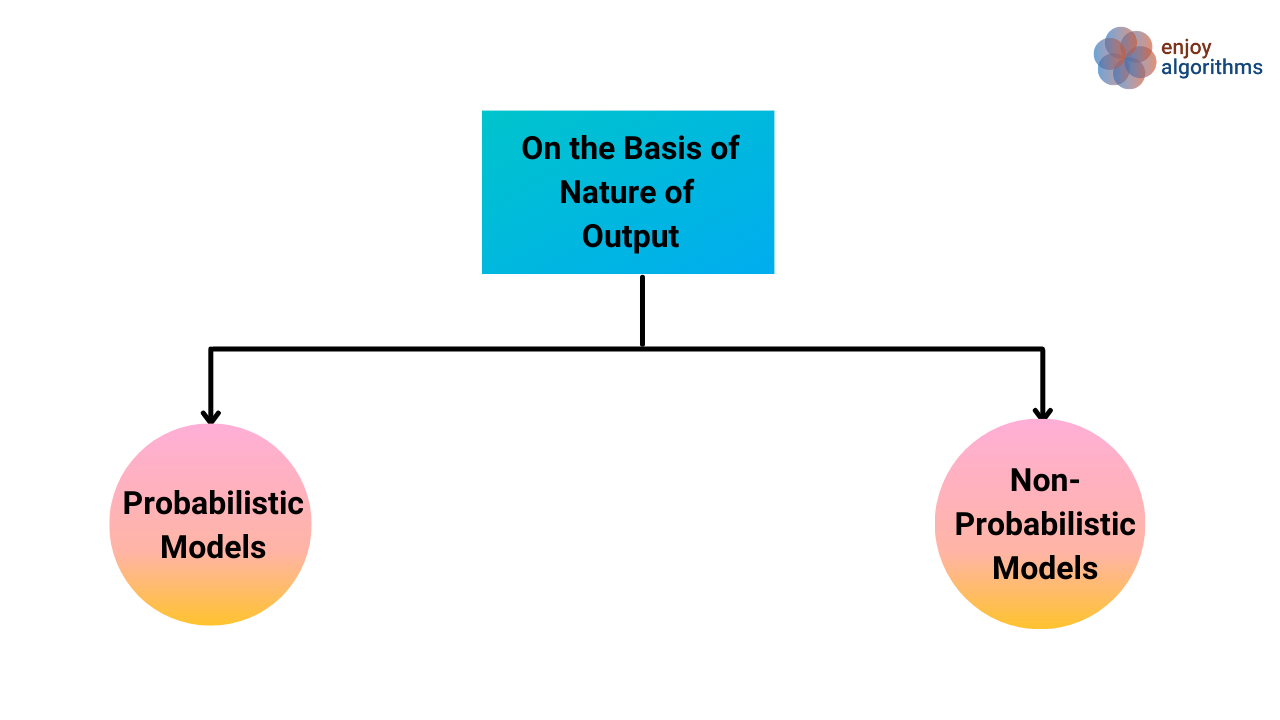1. Probabilistic Models:- It gives the output in the form of probabilities, reflecting the prediction's confidence. For example, the classification problem is relevant to probabilistic models as algorithms predict the label with a certain confidence.
E.g., Suppose our model looks into one picture and says it is 60% sure that a dog is present in that picture. CNN Algorithm is one of the examples in this category.
2. Non-Probabilistic Models:- This model predicts but does not give any measure to know the quality of prediction. But there will be external methods to know the error between the predicted value and the actual value. Decision Tree and SVM are some examples that lie under this category.

### Critical questions to explore

Question 1: On what basis, the same model can be classified as a supervised model and a classification model?
Question 2: What is the difference between supervised and unsupervised learning?
Question 3: What is the difference between classification and clustering?
Question 4: What makes Reinforcement Learning different from supervised, unsupervised and semi-supervised learning?
Question 5: How Deep-Learning and Neural Networks are different?

### Conclusion:-

In this article, we classified machine learning models on the basis of five different categories. The purpose of this article was to make learners aware about all the classifications of machine learning. Later the detailed descriptions about "supervised and unsupervised learning algorithms" and "classification and regression" problems will be presented.

### References:-

1. Machine Intelligence by Suresh Samudrala

### Enjoy Thinking, Enjoy Machine Learning, Enjoy Algorithms!

Subscribe to get well-designed content on data structures and algorithms, machine learning, system design, oops, and mathematics. enjoy learning!

### We Welcome Doubts and Feedback!